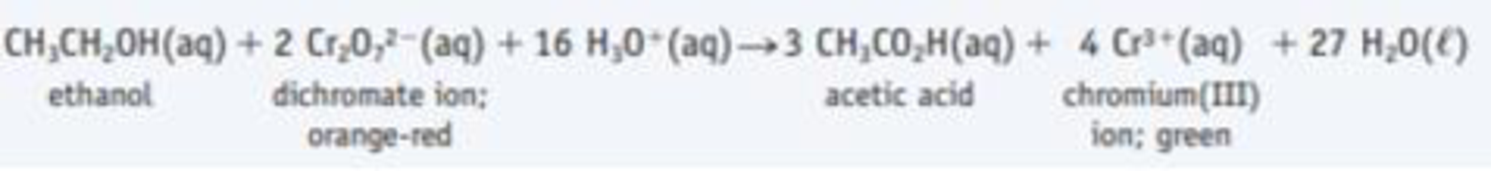Chapter 3.8, Problem 3.9CYU

Chapter
Section
Textbook Problem

The following reaction is used in a device for testing the breath of a person for the presence of ethanol. Identify the oxidizing and reducing agents, the substance oxidized, and the substance reduced.3Interpretation Introduction

Interpretation:

Oxidizing and reducing agents, the substance oxidized and the substance reduced in the given chemical reaction should be identified.

Concept introduction:

An oxidizing agent gains the electrons and is reduced in a chemical reaction and it is electron acceptor.  A reducing agent loses electrons and is oxidized in a chemical reaction and it is electron donor.  The oxidation state is called as oxidation number, which describes degree of oxidation (loss of electrons) of an atom in a chemical compound.  Theoretically, the oxidation state is positive, negative or zero.

Explanation

The given reaction is shown below,

The given compound is Cr2O72- and which is not a neutral compound.  Therefore the overall charge is -2.

The oxidation number of oxygen is -2, therefore the oxidation state of  Cr in Cr2O72- is given below,

Net charge on Cr2O72- is equal to sum of the oxidation number of seven oxygen atoms.

Therefore,

Net charge = Number of oxidation state of oxygen atom -2 = (2x)x

Still sussing out bartleby?

Check out a sample textbook solution.

See a sample solution

The Solution to Your Study Problems

Bartleby provides explanations to thousands of textbook problems written by our experts, many with advanced degrees!

Get Started

The theory that vitamin C prevents or cures colds is well supported by research. T F

Nutrition: Concepts and Controversies - Standalone book (MindTap Course List)

What is the structure and function of a chloroplast?

Biology: The Dynamic Science (MindTap Course List)

List the three major stages in protein synthesis.

Chemistry for Today: General, Organic, and Biochemistry